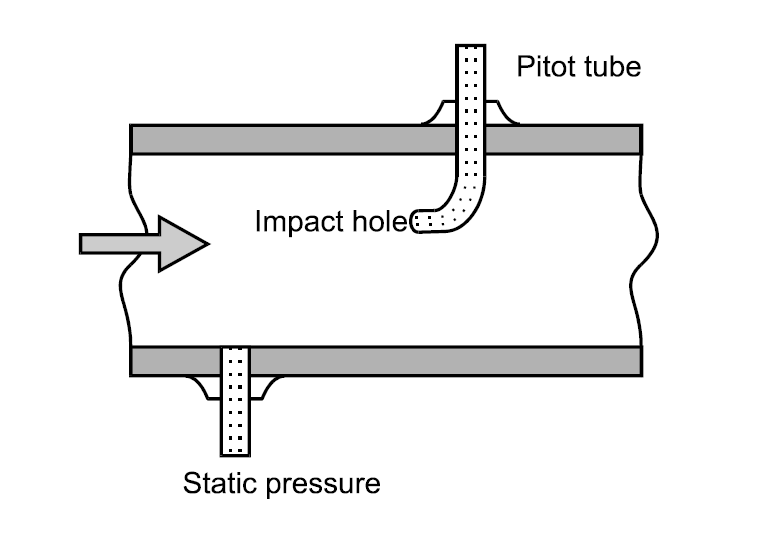Pitot tube is a device used to measure the fluid flow measurement invented by Henri Pitot, a French engineer in 18th century.It is widely to measure the airspeed of aircrafts, speedboat speed and for fluid flow measurement in industrial application. Pitot tube measures the pressure point in contact.

### Principle:

The pitot tube is a differential pressure measuring device.The pitot tube installed in the flow stream measures the direct pressure at the contact pitot tube hole and a second measurement is required, being of static pressure. The difference between the two measurements gives a value for dynamic pressure. The flow rate, like other devices, is calculated from the square root extractor of the pressure.

In a pitot-static tube, the flow Q is given by:

Q = K√(pS − pi)

where K is a constant, pS is the static pressure, and pI is the impact pressure.

## Construction and Working:

The pitot meter consists of a tube pointing directly toward the flow. The fluid enters through the impact hole and there can one or two other holes in the pitot tube, which are the static pressure source.

For a simple pitot tube (shown in fig:1) we should arrange one another pressure sensing element to measure the static pressure. The axis of tube measuring the static pressure should be perpendicular to the boundary and free from burrs so that the boundary is smooth.

The pitot-static tube that having the static pressure inlet is shown below.

A pitot tube is a simple round cylinder with one end opened with a small hole and other end enclosed. The fluid flowing through the pipeline enters the pitot tube and rest there. There is another chamber within the pitot tube filled with fluid with static pressure. A diaphragm separates both the chambers.

The differential pressure is measured between both the pressures gives the dynamic pressure. The difference in level between the liquid in the tube and the free surface becomes the measure of dynamic pressure. The flow rate, like other devices, is calculated from the square root of the pressure.

In calculating the flow rate from the pressure, the calculation is dependent on such factors as tube design and the location of the static tap. The Pitot-static probe incorporates the static holes in the tube system to eliminate this parameter.

Measuring the static pressure and the impact pressure are connected to the proper differential pressure meter for the determination of flow velocity and thus the flow rate.